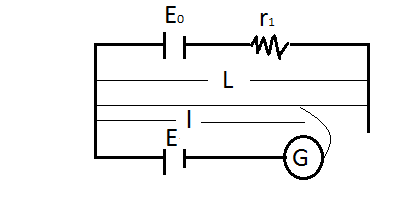# A Potentiometer Wire Of Length L And A Resistance R Are Connected In Series With A Battery Of E.M.F. E0 And A Resistance R1. An Unknown E.M.F. E Is Balanced At A Length L Of The Potentiometer Wire. The E.M.F. E Will Be Given By.The current through the potentiometer wire is

$I = \frac{E_{0}}{r + r_{1}}$

The potential difference across the wire is

$V = Ir = \frac{E_{0}r}{r + r_{1}}$

The potential gradient along the potentiometer wire is

$k = \frac{V}{L} = \frac{E_{0}r}{r + r_{1}L}$

E is balanced against length l of the potentiometer wire

$kl = \frac{E_{0}r}{r + r_{1}L}$

Therefore, the unknown e.m.f. is$\frac{E_{0}r}{r + r_{1}L}$

Explore more such questions and answers at BYJU’S.